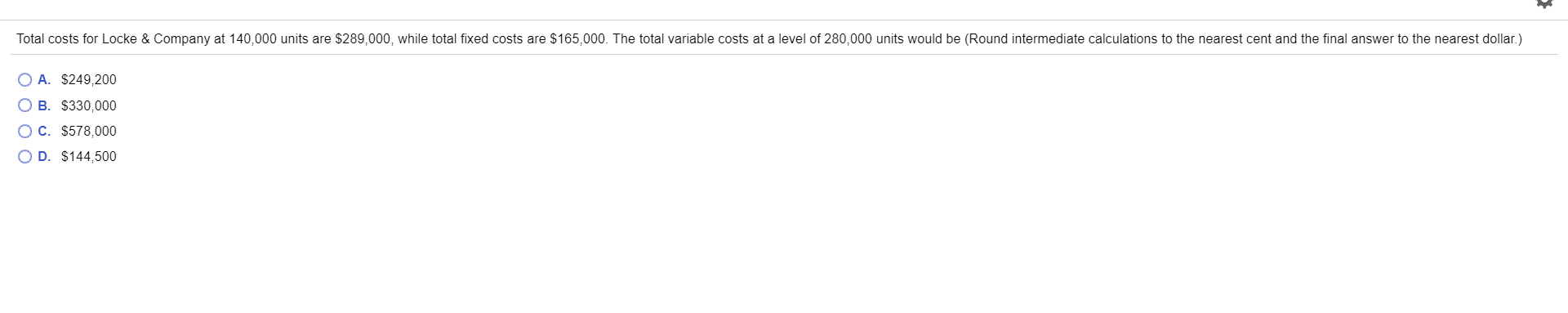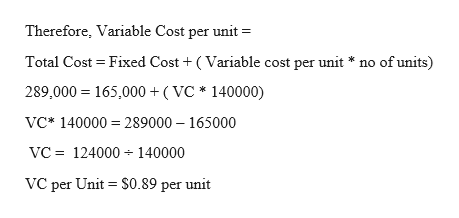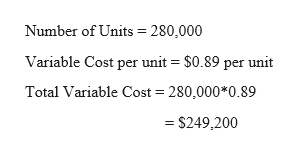# Total costs for Locke & Company at 140,000 units are \$289,000, while total fixed costs are \$165,000. The total variable costs at a level of 280,000 units would be (Round intermediate calculations to the nearest cent and the final answer to the nearest dollar.)O A. \$249,200B. \$330,000C. \$578,000O D. \$144,500

Questionhelp_outlineImage TranscriptioncloseTotal costs for Locke & Company at 140,000 units are \$289,000, while total fixed costs are \$165,000. The total variable costs at a level of 280,000 units would be (Round intermediate calculations to the nearest cent and the final answer to the nearest dollar.) O A. \$249,200 B. \$330,000 C. \$578,000 O D. \$144,500 fullscreen
check_circleExpert Solution
Step 1

Cost incurred to produce and sell a product consist of 2 components, variable cost, and fixed cost.

Variable cost is the cost that changes with the change in production levels. It is directly proportional to the units produced.

Fixed cost is the other indirect cost incurred by the business. These are fixed in nature and do not change with the change in production level.

Step 2

Given Information:

Number of Units = 140,000

Total Cost = \$289,000

Fixed Cost = \$165,000help_outlineImage TranscriptioncloseTherefore, Variable Cost per unit Total Cost Fixed Cost (Variable cost per unit * no of units) 289,000 165,000 ( VC* 140000) VC* 140000 289000 165000 VC 124000 140000 VC per Unit \$0.89 per unit fullscreen
Step 3

Computation of Variable co...help_outlineImage TranscriptioncloseNumber of Units 280,000 Variable Cost per unit = \$0.89 per unit Total Variable Cost 280,000*0.89 \$249,200 fullscreen

### Want to see the full answer?

See Solution

#### Want to see this answer and more?

Solutions are written by subject experts who are available 24/7. Questions are typically answered within 1 hour*

See Solution
*Response times may vary by subject and question
Tagged in